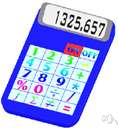# expected value

(redirected from Mathematical expectancy)
Also found in: Thesaurus, Financial, Encyclopedia.

## ex·pect·ed value

(ĭk-spĕk′tĭd)
n.
1. A quantity expressing a typical or average value of a random variable.
2. The sum (for discrete variables) or integral (for continuous variables) of the product of a random variable with its probability density function, over its range of values.
 Noun 1expected value - the sum of the values of a random variable divided by the number of valuesstatistics - a branch of applied mathematics concerned with the collection and interpretation of quantitative data and the use of probability theory to estimate population parametersmean, mean value - an average of n numbers computed by adding some function of the numbers and dividing by some function of n